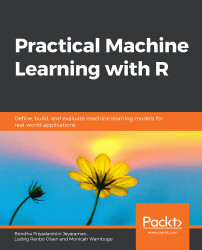•#### Practical Machine Learning with R#### Overview of this book

With huge amounts of data being generated every moment, businesses need applications that apply complex mathematical calculations to data repeatedly and at speed. With machine learning techniques and R, you can easily develop these kinds of applications in an efficient way. Practical Machine Learning with R begins by helping you grasp the basics of machine learning methods, while also highlighting how and why they work. You will understand how to get these algorithms to work in practice, rather than focusing on mathematical derivations. As you progress from one chapter to another, you will gain hands-on experience of building a machine learning solution in R. Next, using R packages such as rpart, random forest, and multiple imputation by chained equations (MICE), you will learn to implement algorithms including neural net classifier, decision trees, and linear and non-linear regression. As you progress through the book, you’ll delve into various machine learning techniques for both supervised and unsupervised learning approaches. In addition to this, you’ll gain insights into partitioning the datasets and mechanisms to evaluate the results from each model and be able to compare them. By the end of this book, you will have gained expertise in solving your business problems, starting by forming a good problem statement, selecting the most appropriate model to solve your problem, and then ensuring that you do not overtrain it.Free Chapter
An Introduction to Machine LearningData Cleaning and Pre-processingFeature EngineeringIntroduction to neuralnet and Evaluation MethodsLinear and Logistic Regression ModelsUnsupervised LearningAppendix## Advanced Operations on Data Frames

In the previous chapter, we performed a number of operations on data frames, including rbind(). There are many more operations that can be performed on data frames, which are very useful while preparing the data for the model. The following exercises will describe these operations in detail and illustrate them through their corresponding implementation in R:

• The order function: The order function is used to sort a data frame. We can specify ascending or descending order using the "-" symbol.
• The sort function: The sort function can also be used to sort the data. The order can be specified as "decreasing=TRUE" or "decreasing"="FALSE".
• The rank function: The rank function is used to rank the values in the data in a numerical manner.

Sorting, ordering, and ranking are operations that act as techniques to identify outliers. Outliers are values that are either too big or too small and do not fit in the value...